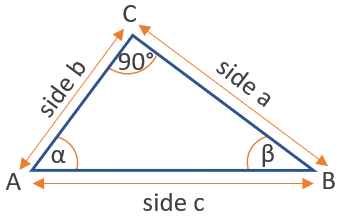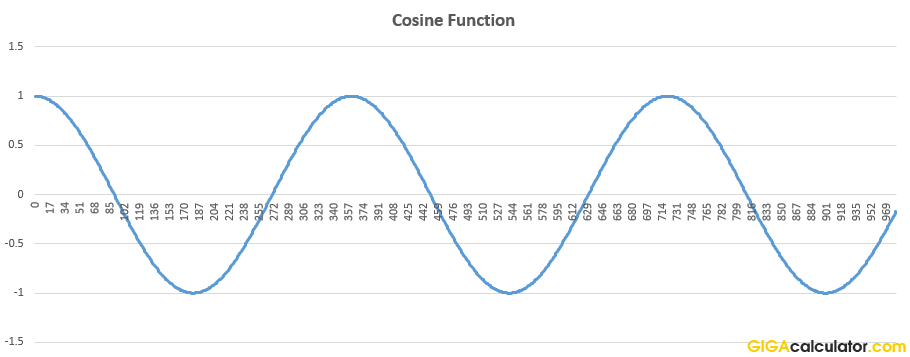# Cosine Calculator

Use this cos calculator to easily calculate the cosine of an angle given in degrees or radians. The cosine is encountered in geometry problems involving right triangles, circles, and others where cos(x) can be calculated.

### Calculation results

0 cos(90) =
Share calculator:

Embed this tool:
get code

## The Cosine function ( cos(x) )

The cosine is a trigonometric function of an angle, usually defined for acute angles within a right-angled triangle as the ratio of the length of the adjacent side to the hypotenuse. It is the complement to the sine. In the illustration below, cos(α) = b/c and cos(β) = a/c.Since cos(α) = b/c, from this definition it follows that the cosine of any angle is always less than or equal to one, and it can take negative values. The cosine of a 90-degree angle is equal to zero, since in order to calculate it we would need a triangle with two 90-degree angles, which is the definition of a straight line. As the third side of the triangle does not exist (length is 0), the cosine equals zero (0 divided by the length of the hypotenuse equals 0). You can use this cosine calculator to verify this.

A commonly used law in trigonometry which is trivially derived from the cosine definition is the law of cosines: c2 = a2 + b2 - 2ab·cosγ

## Related trigonometric functions

The reciprocal of cosine is the secant: sec(x), sometimes written as secant(x), which gives the ratio of the length of the hypotenuse to the length of the side opposite to the angle.

The inverse of the cosine is the arccosine function: acos(x) or arccos(x), which takes values between 0 and 180 degrees. It is useful for finding an angle x when cos(x) is known.

## How to calculate the cosine of an angle?

Our cosine calculator supports input in both degrees and radians, so once you have measured the angle, or looked up the plan or schematic, you just input the measurement and press "calculate". This is how easy it is.

If the angle is unknown, but the lengths of the adjacent side and the hypotenuse of a right angle triangle are given, then calculating the cosine can be done by dividing the adjacent side by the hypotenuse (side c as per the figure above). For example, if one needs to find cot(α) and it is known that the adjacent side b = 6 and c = 20, then cos(α) = 6 / 20 = 0.3.

## Applications of the cosine function

The cosine function can be used to model periodic phenomena in physics, biology, social sciences, etc. A more practical example is if you want to cut down a tree and you know its height and want to know how far from it you are currently standing, use the tan function.

JPEG, a very common image compression algorithm, uses so-called "discrete cosine transform" function (DCT) to express finite sequences of data points as sums of cosine functions with different oscillation frequencies. A modified version is employed as the basis for the popular audio compression codec MP3, as well as AAC, Vorbis, and WMA. MPEG and DV are also based on similar calculus.Above: a wave generated using the cosine function. A cosine wave is the mirror image of a sine wave.

Table of common cosine values:

Common values of the cosine function
0 1
30° π/6 0.866025
45° π/4 0.707107
60° π/3 0.50
90° π/2 0
120° 2π/3 -0.50
135° 3π/4 -0.707107
150° 5π/6 -0.866025
180° π -1

#### Cite this calculator & page

If you'd like to cite this online calculator resource and information as provided on the page, you can use the following citation:
Georgiev G.Z., "Cosine Calculator", [online] Available at: https://www.gigacalculator.com/calculators/cosine-calculator.php URL [Accessed Date: 28 Sep, 2023].